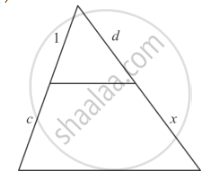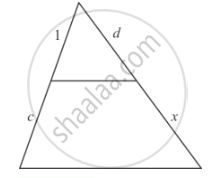Advertisement Remove all ads

# In Each of the Figures [(I)-(Iv)] Given Below, a Line Segment is Drawn Parallel to One Side of the Triangle and the Lengths of Certain Line-segment Are Marked. Find the Value of X in Each of the - Mathematics

Sum

In each of the figures [(i)-(iv)] given below, a line segment is drawn parallel to one side of the triangle and the lengths of certain line-segment are marked. Find the value of x in each of the following :Advertisement Remove all ads

#### Solution

In each of the figure, we have to find the value of x1/(1+c)=d/(d+x)

By cross multiplication on both sides, we get

1xx(d+x)=dxx(1+c)

d+x=d+dc

x=d+dc-d

x= cancel(d)+dc-cancel(d)

x=dc

Hence the value of  x is dc

Concept: Triangles Examples and Solutions
Is there an error in this question or solution?
Advertisement Remove all ads

#### APPEARS IN

RD Sharma Class 10 Maths
Chapter 7 Triangles
Q 1.1 | Page 123
Advertisement Remove all ads

#### Video TutorialsVIEW ALL 

Advertisement Remove all ads
Share
Notifications

View all notifications

Forgot password?
Course